## Analogy

#### Analogy

Direction: In following questions, select the related figure from the given alternatives.

1. Find the question mark ? figure from answer figure.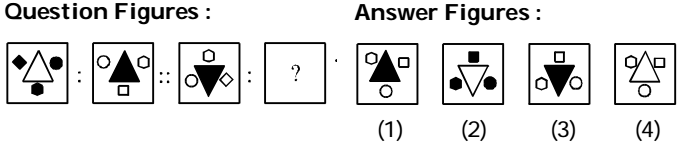1. The main design becomes shaded and other three small designs become white and moves in anticlockwise and the quadrilateral rotates through 45°.

##### Correct Option: B

From first figure to second figure the main design becomes shaded and other three small designs become white and moves in anticlockwise and the quadrilateral rotates through 45°.

1. Find the question mark ? figure from answer figure.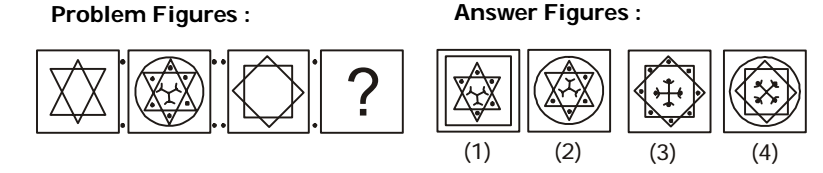1. From first figure to second figure one dot is added to every sector of the design and three line segments with curves are introduced in the centre.

##### Correct Option: C

From first figure to second figure one dot is added to every sector of the design and three line segments with curves are introduced in the centre.

1. Find the question mark ? figure from answer figure.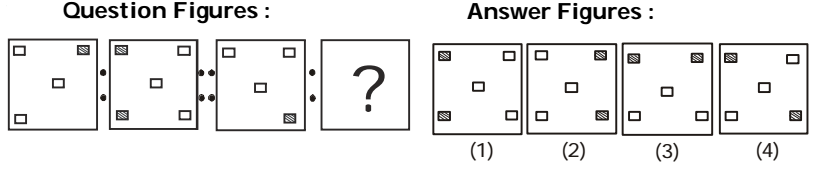1. From first figure to second figure the white square moves to the opposite side and the shaded square also moves to the opposite side and then it duplicates.

##### Correct Option: C

From first figure to second figure the white square moves to the opposite side and the shaded square also moves to the opposite side and then it duplicates.

1. Find the question mark ? figure from answer figure.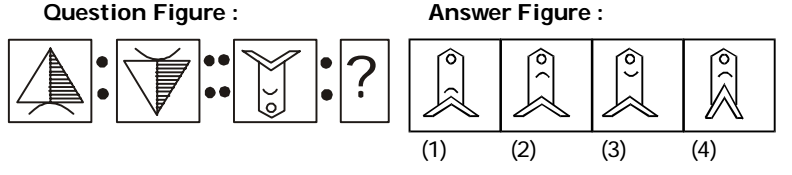1. The second figure is water image of the first figure.

##### Correct Option: B

The second figure is water image of the first figure.

1. Find the question mark ? figure from answer figure.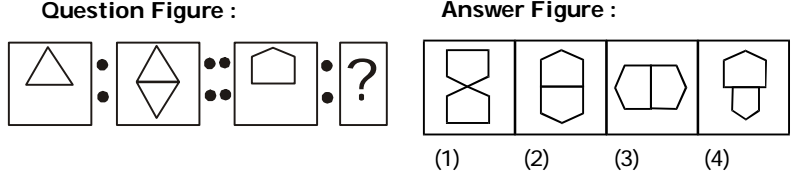1. From first figure to second figure a similar but inverted figure is added.

##### Correct Option: B

From first figure to second figure a similar but inverted figure is added.